# Introduction - Towards Discoverability

Results are most impactful when they are reproducible, understandable, and discoverable; Analysts cannot incorporate a finding into their understanding of a particular dataset or question unless they know that result exists. This can be difficult within the status quo, where results are often sent directly to collaborators on a particular project, and then (hopefully) archived - often in a location and manner specific to the analyst who generated them.

Results are discoverable on the other hand, when there is a reasonable mechanism by which anyone with appropriate access permissions can discover the existance of - and locate - them. The searching party might be a new collaborator getting up to speed on a project, a scientist working in the same space and trying to determine what has already been done, or even the analyst themselves looking for a result generated months or years previous.

The `trackr` package seeks to improve the discoverability of results by both recording the existence of (and in some cases object representing) R-based results in a customizable database, and annotating those rescords with automatically inferred metadata about those results. These annotations power the ability to search for and find records of particular results, or classes thereof, whether or not the seeker knew of them beforehand.

# Dependency installation check

To run all examples and vignettes, the following packages should be installed:

• From CRAN: `ggplot2`, `lattice`, `proto`, `digest`, `png`, `httr`, `MASS`, `MEMSS`, `mlmRev`, `devtools`, `CodeDepends`, `rsolr`
• From Bioconductor: `BiocGenerics`

# Setup

## Use temporary trackr backend

By default trackr will write to a permanent default JSON backend which lives at `~/.trackr/objdb.json`. For the purposes of this vignette, we point it at a temporary one so the vignette does not create permanent files as it is running.

Users will generally not need to run the code below, though they may choose to utilize a non-default backend in which case they will need to specify that in their session.

``````library(trackr)

tdb = TrackrDB(backend = JSONBackend(file = file.path(tempdir(), "objdb.json")),
img_dir = file.path(tempdir(), "trackr_img_dir"))

defaultTDB(tdb)``````
``````## An object of class "TrackrDB"
## Slot "opts":
## An object of class "TrackrOptions"
## Slot "insert_delay":
##  0
##
## Slot "img_dir":
##  "/var/folders/79/l_n_5qr152d2d9d9xs0591lh0000gn/T//Rtmpc86C3u/trackr_img_dir"
##
## Slot "img_ext":
##  "png"
##
## Slot "backend_opts":
## list()
##
##
## Slot "backend":
## Reference class object of class "JSONBackend"
## Field "docs":
## DocList (0x0)
## Field ".file":
##  "/var/folders/79/l_n_5qr152d2d9d9xs0591lh0000gn/T//Rtmpc86C3u/objdb.json"
## Field "file":
##  "/var/folders/79/l_n_5qr152d2d9d9xs0591lh0000gn/T//Rtmpc86C3u/objdb.json"``````

## Create some example plots

Here we create a number of plots - ggplot2, lattice, and base - which we will use throughout the demonstration. The details of these plots themselves is not improtant, but we use many different datasets and plotting methodologies in order to illustrate the different avenues for discoverability enabled by trackr’s automatic annotations.

``````library(ggplot2)
library(lattice)

## examples from
##      http://www.cookbook-r.com/Graphs
##      https://learnr.files.wordpress.com/2009/08/latbook.pdf
##      http://docs.ggplot2.org/current/index.html
##      http://lmdvr.r-forge.r-project.org/figures/figures.html

## modified version of Figure 1.1 in
## http://lmdvr.r-forge.r-project.org/figures/figures.html
data(Chem97, package = "mlmRev")
pl <- histogram(~gcsescore | factor(score),
data = Chem97, main="Lattice Histogram of gcsescore", sub="conditioned on score")
pl``````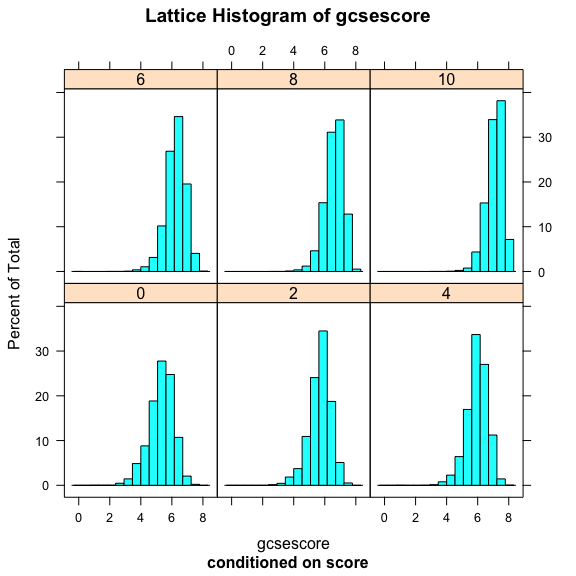``````## and a modified version of its ggplot2 counterpart, from
## https://learnr.files.wordpress.com/2009/08/latbook.pdf
pg <- ggplot(Chem97, aes(gcsescore)) +
geom_histogram(binwidth = 0.5) +
facet_wrap(~score) +
ggtitle(expression(atop("ggplot2 Histogram of gcsescore", atop("facetted by score")))) +
theme_bw()
pg``````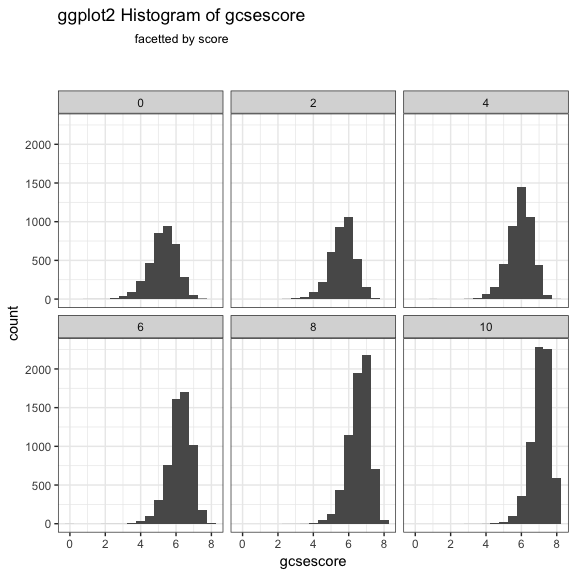``````## modified version of a graphic available here:
## http://docs.ggplot2.org/current/scale_hue.html
set.seed(620)
dsamp <- diamonds[sample(nrow(diamonds), 1000), ]
d <- ggplot(dsamp, aes(carat, price, colour = clarity)) +
ggtitle("Diamond price by carat and clarity") +
geom_point() + theme_bw()
d``````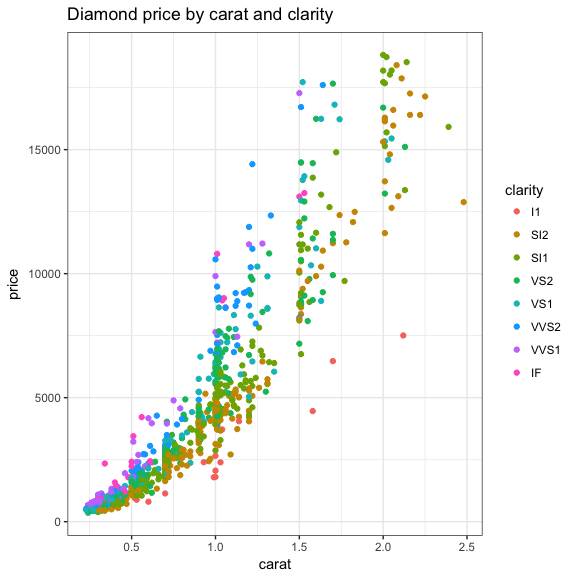``````## modified version of a graphic available here:
## http://docs.ggplot2.org/current/stat_density2d.html
data(geyser, package = "MASS")
m <- ggplot(geyser, aes(x = duration, y = waiting)) +
geom_point() + geom_density2d() +
xlim(0.5, 6) + ylim(40, 110) +
xlab("Eruption time in min") + ylab("Waiting time in min") +
ggtitle("Eruption length and waiting time") +
coord_trans(y="log10") + theme_bw()
m``````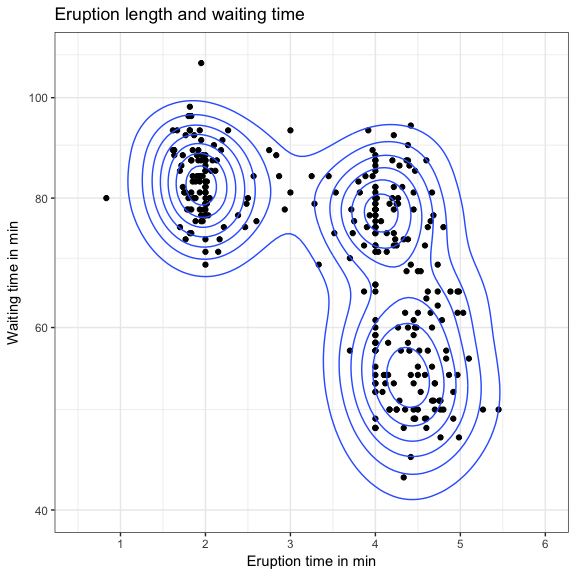``````## modified version of Figure 2.1 in
## http://lmdvr.r-forge.r-project.org/figures/figures.html
data(Oats, package = "MEMSS")
tp1.oats <- xyplot(yield ~ nitro | Variety + Block, data = Oats,
type = 'o',
xlab = "Nitrogen conc (cwt/acre)",
ylab = "Yield (bushels/acre)",
main = "Yield by nitrogen | variety + block")
tp1.oats``````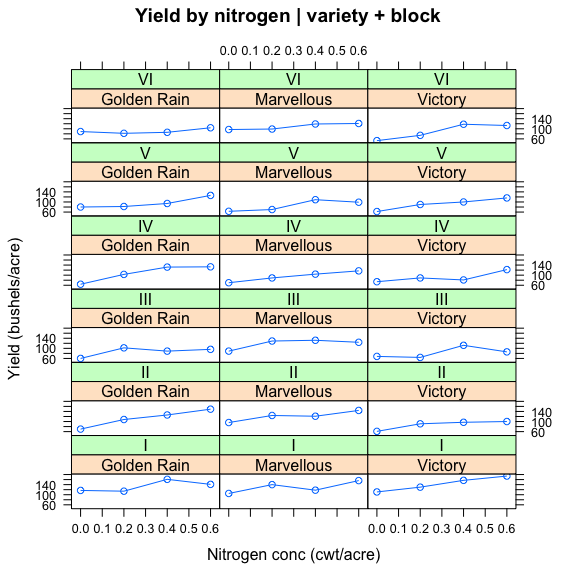``````## and a modified version of its ggplot2 counterpart, from
## https://learnr.files.wordpress.com/2009/08/latbook.pdf
pg.oats <- ggplot(Oats, aes(nitro, yield)) +
geom_line() + geom_point(shape = 'o') +
facet_grid(Block ~ Variety) +
xlab("Nitrogen concentration (cwt/acre)") +
ylab("Yield (bushels/acre)") +
ggtitle("Yeild by nitrogen, facetted by variety and block") + theme_bw()
pg.oats``````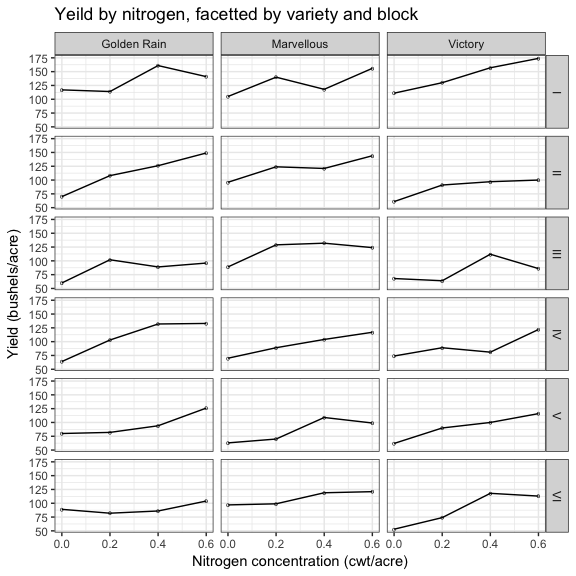``````## Figure 1.2 in
## http://lmdvr.r-forge.r-project.org/figures/figures.html
pl2 <- densityplot(~ gcsescore | factor(score), data = Chem97,
plot.points = FALSE, ref = TRUE, main = "Density of gcsescore", subtitle= "conditioned on score")
pl2``````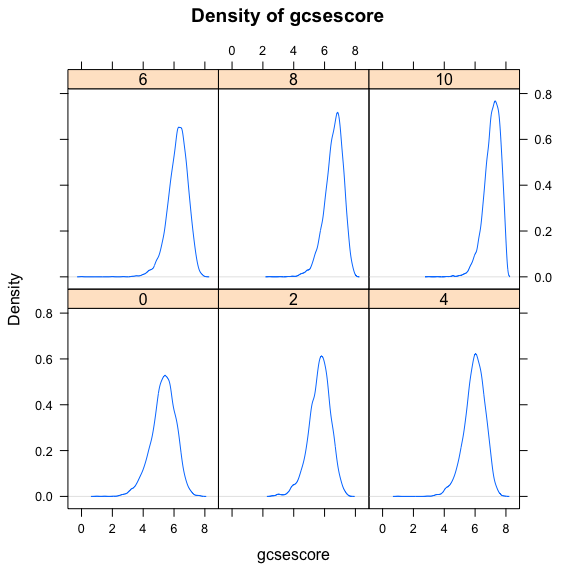``````## and a modified version of its ggplot2 counterpart, from
## https://learnr.files.wordpress.com/2009/08/latbook.pdf
pg2 <- ggplot(Chem97, aes(gcsescore)) +
stat_density(geom = "path", position = "identity") +
facet_wrap(~score) +
ggtitle(expression(atop("Density of gcsescore", atop(" facetted by score")))) + theme_bw()
pg2``````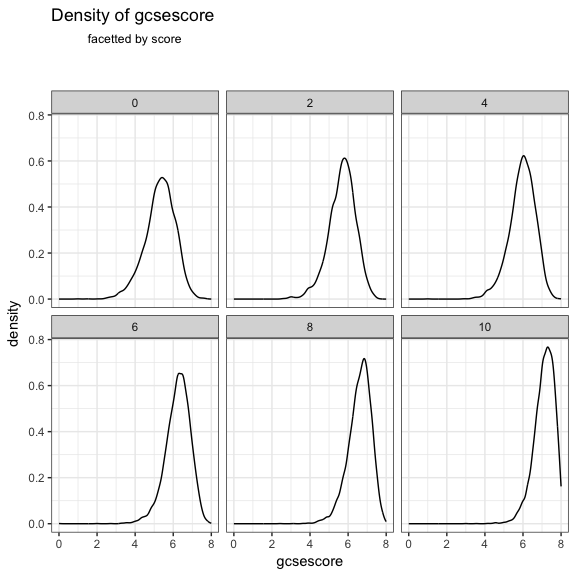``````## a modified version of a graphic available here:
## http://docs.ggplot2.org/current/coord_trans.html
df.abc <- data.frame(a = abs(rnorm(26)), letters)
pg3 <- ggplot(df.abc, aes(a, letters)) +
geom_point() + coord_trans(x = "sqrt") +
annotate("text", x = 1.25, y = 5, label = "Some text") +
ggtitle("Simulated normal data vs letters") +
theme_bw()
pg3``````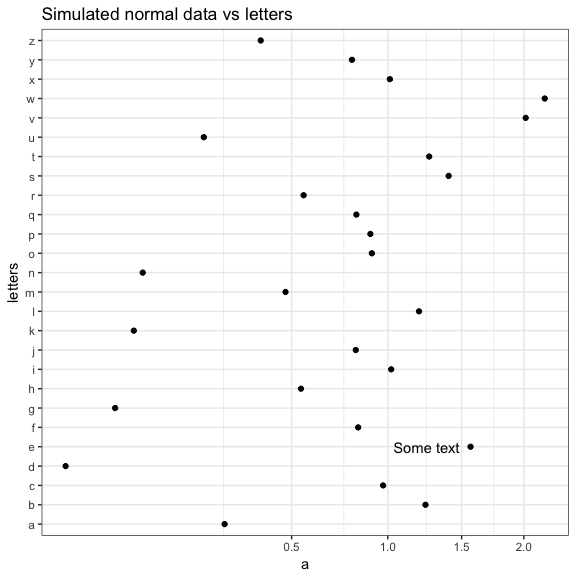``````## and a lattice version to match
pl3 <- xyplot(letters ~ a, data = df.abc,
panel = function(x, y,...) {
panel.xyplot(x, y,...)
panel.text(1.25, 5, labels = "Some text")
},
main = "Simulated data vs letters v2"
)
pl3``````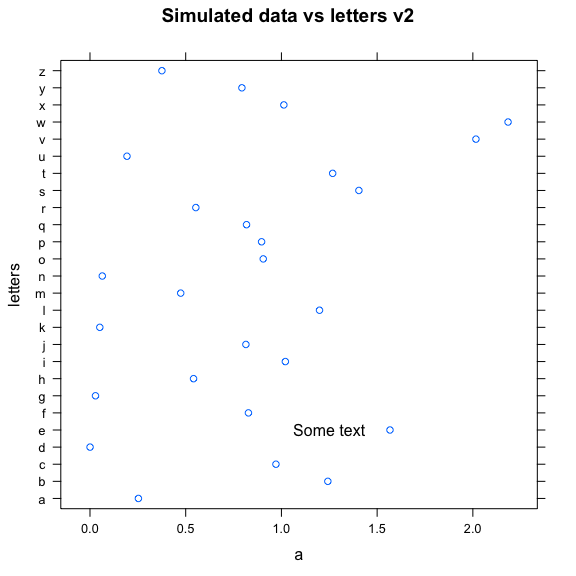``````## Figure 1.3 in
## http://lmdvr.r-forge.r-project.org/figures/figures.html
pl4 <- densityplot(~ gcsescore, data = Chem97, groups = score,
plot.points = FALSE, ref = TRUE,
auto.key = list(columns = 3),
main = "Densities of gcsescore by score")
pl4``````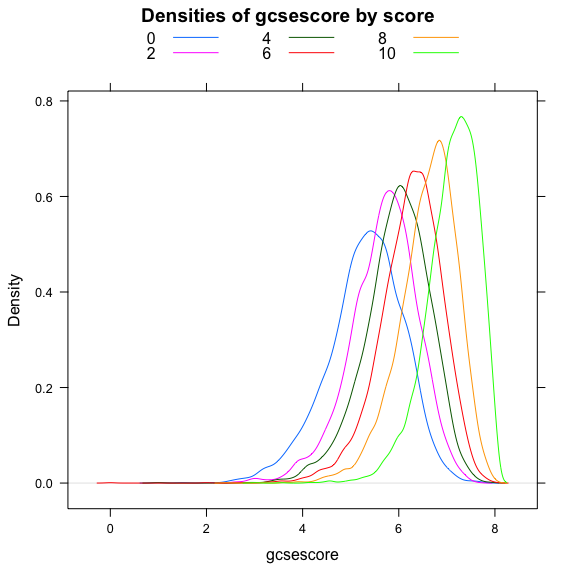``````## and a modified version of its ggplot2 counterpart, from
## https://learnr.files.wordpress.com/2009/08/latbook.pdf
pg4 <- ggplot(Chem97, aes(gcsescore)) +
stat_density(geom = "path", position = "identity",
aes(colour = factor(score))) +
ggtitle("Densities of gcsescore by score 2") + theme_bw() +
theme(legend.position = 'top')
pg4``````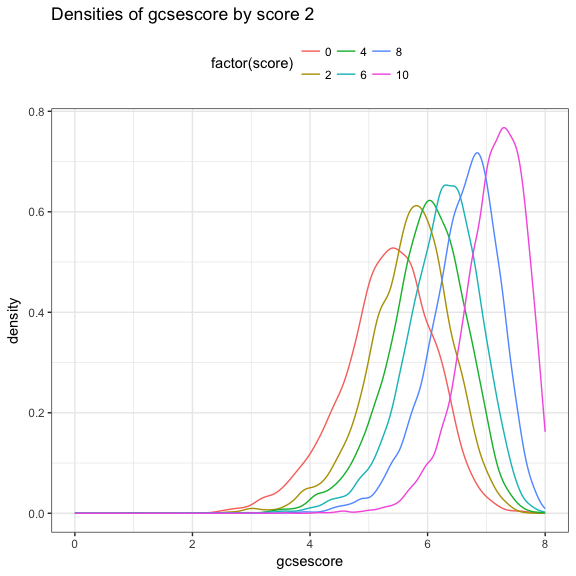``````## a custom example, with both lattice and ggplot2 versions
x = sample(1:5, 1000, replace=TRUE)
y = rnorm(1000, x, sd = 1/sqrt(x))
lp = densityplot(~y, groups = x,
auto.key=list(space="right", title="x", points=TRUE), main = "More simulated data")
lp``````